# Interactive Random Walk Demonstrations

This page shows small excerpts of what you can see if you download the corresponding CDF file. This is "Computable Document Format" of Wolfram. I made the files with Mathematica. Wolfram no longer provides a plugin, so in order to see more, you will need to download the CDF file. If you already have either Mathematica or the free Wolfram CDF Player, then you need nothing else.

 (If you already have Mathematica, you do not need this.)

Here we can play with 1-dimensional random walks, thought of as games where winning and losing has probability 1/2 each, and we keep track of the number of wins minus the number of losses. You may be surprised at how infrequently the random walk crosses 0. (You can see one random instance after another by changing "new random case".) This can be quantified by the arc sine law.

The random walk converges to Brownian motion. You can view Brownian motion here. You can zoom Brownian motion at 0 up to a factor of 280. The vertical scale changes with Brownian scaling, that is, the square root of the amount of horizontal zooming. This illustrates the fact that Brownian motion crosses 0 at times arbitrarily close to 0. You can choose to display the functions $\pm\sqrt x$ and $\pm2\sqrt x$ for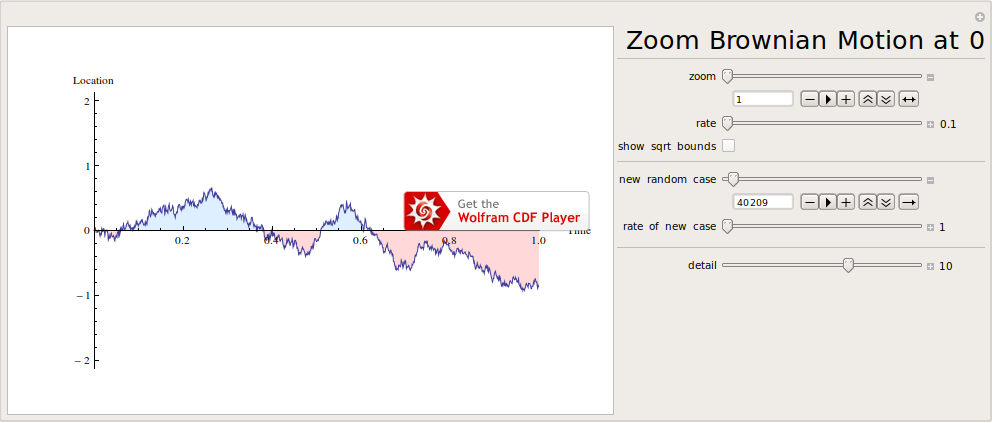Here is a bicycle race. The race lasts 5 laps (which you can change). Each rider has a random forward movement during each small increment of time. All steps are IID and all riders are also IID, unless you choose to make them have different speeds. The "speed diff" is a percent and will be how much faster each rider i is than rider i+1 on average. You can choose to show the average speed of all riders.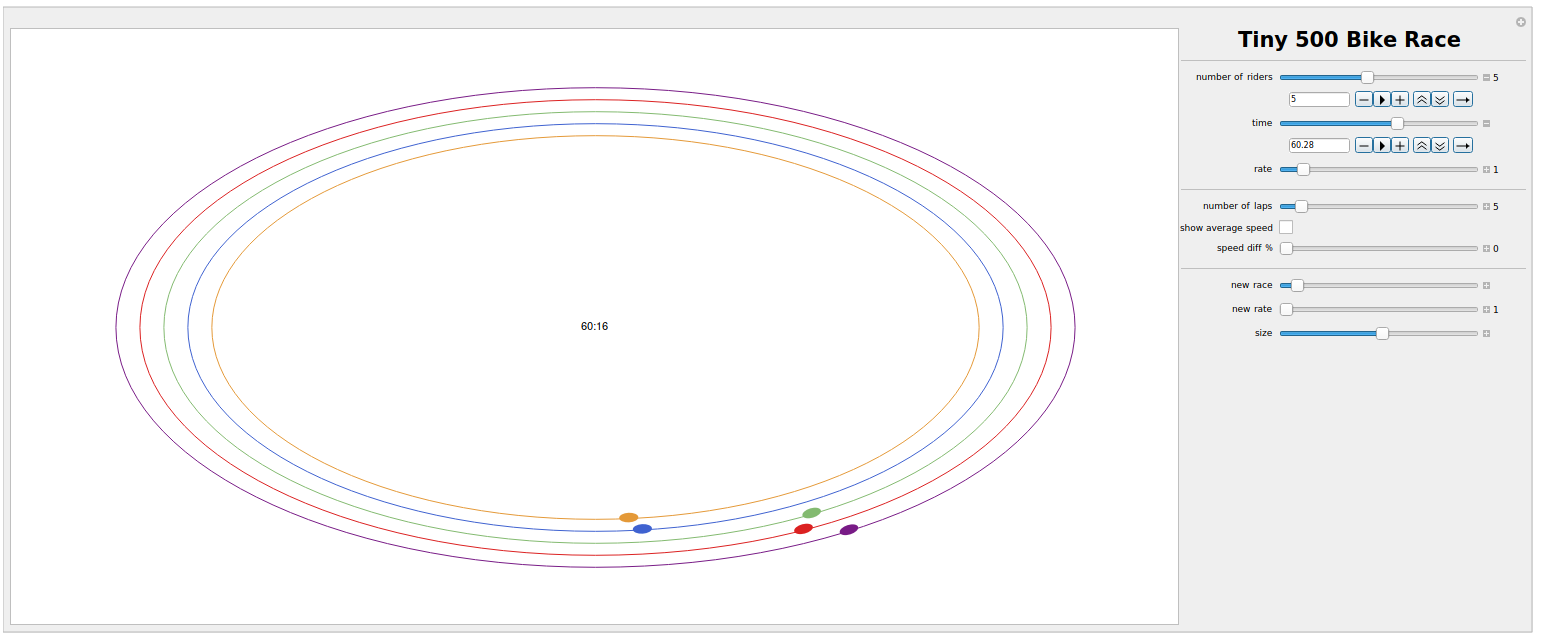The 2-dimensional Brownian motion as Brown viewed it can be seen here:If we have two types of particles, each one performing Brownian motion, we can see them mix. You can choose the rate at which the demo updates; for the fastest possible, make the rate 1000.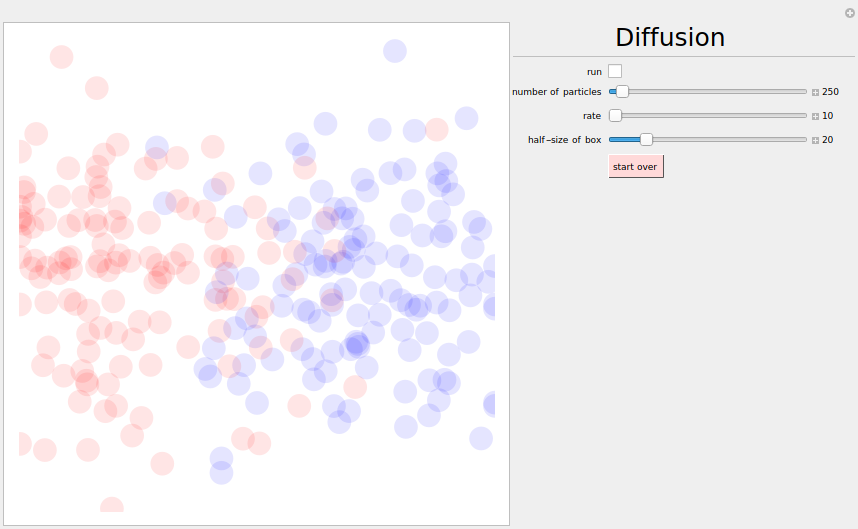'

The next demo allows many more particles, each much smaller, but it can be quite slow with many particles in a large box. You can choose the rate at which the demo updates; for the fastest possible, make the rate 1000. You can change "color clip" in order to see varying levels of detail; the value of "color clip" is the upper limit for the colors, with more particles than this being truncated at the limit. (More precisely, the number of particles of each color is divided by this value, and the result is truncated at 1.)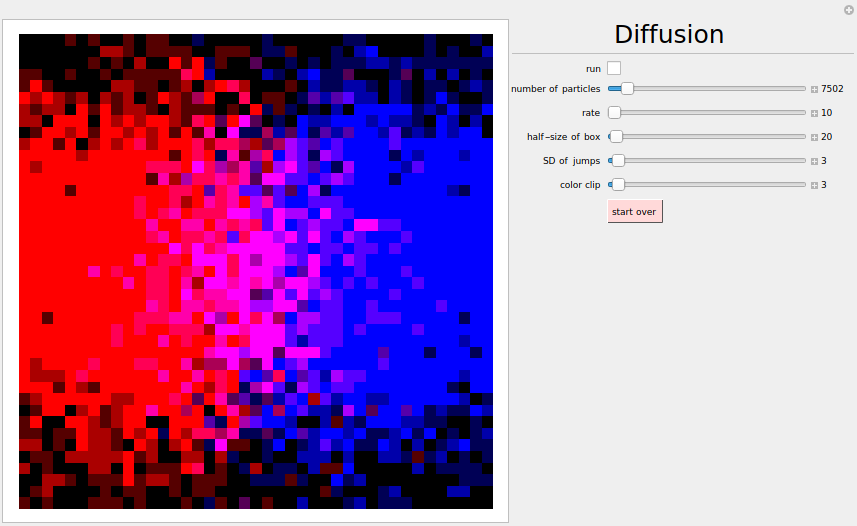However, it still will not look very smooth as in the real world. The real world has so many particles (10 grams of water contains about 6 x 1023 molecules of water, Avogadro's number) that it is essentially the same as continuous. We do not see the discrete nature of the real world. In fact, Einstein in 1905 gave the first quantitative explanation of Brownian motion; this gave firm evidence for the existence of molecules. In the following demo, we show the continuous version of diffusion, which is the expectation of Brownian motion. The first one is for 1 dimension, while the second is for 2 dimensions.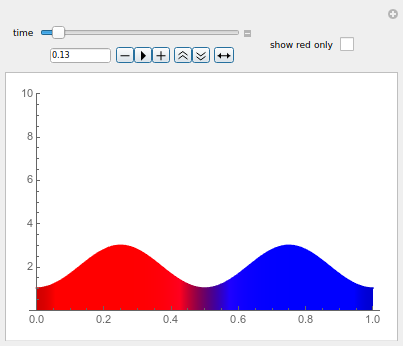The 2-dimensional Brownian motion is a limit of 2-dimensional random walks. In the video, the newest steps are in red, the oldest in blue, and the intermediate ones in green, so that you can see the time aspect of the random walk. Colors are interpolated throughout the walk.

Now we keep track of how many times each site is visited by the random walker (or by many random walkers), either in the whole plane or restricted to just a piece of the square lattice with 2n+1 vertices on each side, where 2n+1 is "half size". This is known as "local time". You can choose the rate at which the demo updates; for the fastest possible, make the rate 1000. You can also display a bar graph showing the number of sites that have a given local time, i.e., the distribution of the local time on the sites. The bar graph also gives the color code for the local time. If the size is very large, the display can get very slow, and then it can abort due to a timeout. If that happens, simply increase the timeout. For some explanation of quantitative phenomena you may observe, see this paper and this paper.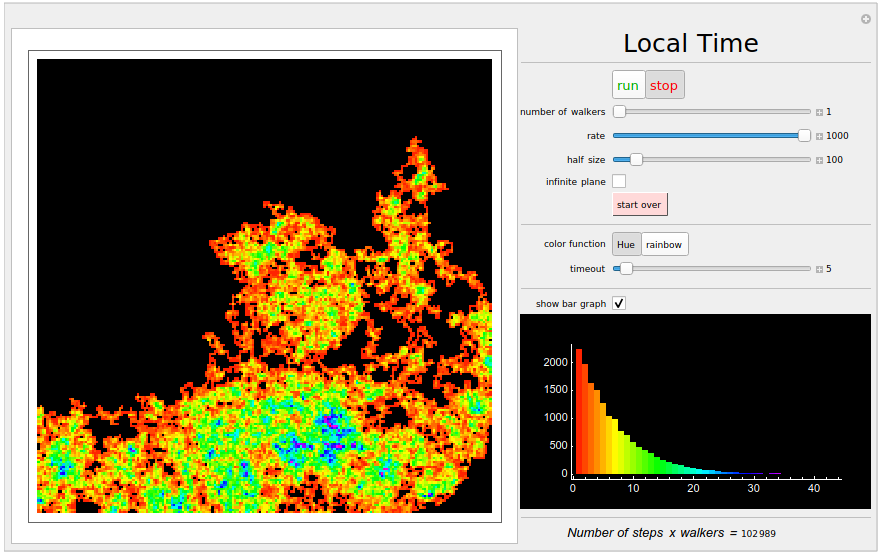Now we restrict random walk to just a piece of the square lattice with n vertices on each side, where n is "size of graph". However, we draw an edge only when the walker arrives at a vertex it has never visited before. The walker will eventually (with probability 1) visit each of the vertices, and at this point, no new edges will be drawn, so we might as well stop. What will we see? Take a look! We got a spanning tree of the graph, i.e., a subset of the edges of the graph such that for every pair of vertices, a and z, of the graph, there is a unique path of edges in the spanning tree that connects a to z. Of course, the particular spanning tree that results is random since the walk is random. What is its distribution? Amazingly, it is uniformly distributed among all possible spanning trees of the graph, i.e., all are equally likely! You can turn this into a maze by increasing the thickness of the edges.Very interesting phenomena occur when we consider lamps at the locations of the random walker. Imagine that a lamplighter must turn on the lamps at all locations, of which there are infinitely many, one at each integer. His home is the origin (0), and all lamps are off during the day. However, as night approaches, he must turn them on. Faced with this impossible task, he gets drunk and thus walks around at random, switching the state of the lamp at random as well. That is, at each time step, he may either walk left 1, walk right 1, or switch the lamp where he is (on to off or off to on). Each of these 3 choices has probability 1/3 each time, as you can see in the demo below.

A state now consists of the location of the lamplighter as well as the status of each lamp (on/off). It turns out that these states form a group: the identity element is where the lamplighter is at 0 and all lamps are off. Only finitely many lamps can be on; each state can be thought of as a sequence of (non-random) instructions to the lamplighter, and group multiplication is found by composing two such sequences. How many group elements are within distance n of the identity? Quite a few: roughly φn, where φ = (1+ $\sqrt5$)/2 ≅ 1.618 is the golden ratio. (Hint: consider only instructions that never move left and observe the Fibonacci numbers appear.) Thus, this lamplighter group has exponential growth. But how fast does the lamplighter move away from the identity? After n steps, he has usually moved around $\sqrt n$ away from 0 and thus there are roughly $\sqrt n$ lamps on within distance roughly $\sqrt n$ (as you can see in the demo). This is at distance only roughly $\sqrt n$ from the identity, so just like random walk on the integers, the distance increases very slowly, with 0 limiting speed (= distance over time).

As morning approaches, the lamplighter realizes he has a reasonable task of turning off all lamps and also the effect of the alcohol begins to wear off. Thus, while still somewhat drunk, he is more likely to make choices that decrease his distance to the identity. In other words, there is some λ > 1 such that each choice that decreases his distance to the identity has weight λ, while all other choices have weight 1. This weighting factor λ increases so that eventually it is infinite and he is no longer drunk. In the demo, λ is called "bias". You can see that when the bias is large, the lamplighter quickly manges his task. What happens when λ is only moderate? It is quite surprising that when 1 < λ < φ, then actually the lamplighter is worse off: his speed is positive! For a proof, see this paper. There is also a surprise when λ < 1, but I won't spoil it for you.

What happens if the lamplighter is walking in 2 dimensions? Similar calculations for the unbiased case (λ = 1) show that again the speed is 0, but in 3 and more dimensions, the speed is positive. This relies on Pólya's theorem.

But what about the biased lamplighter in 2 dimensions? No one knows, except that when λ is smaller than the exponential growth rate, then the biased random walk is transient, while if λ is larger than the growth rate, the biased random walk is recurrent (this holds for general Cayley graphs; see this paper). It would be wonderful to see a simulation, but note that a traveling salesman problem must be solved!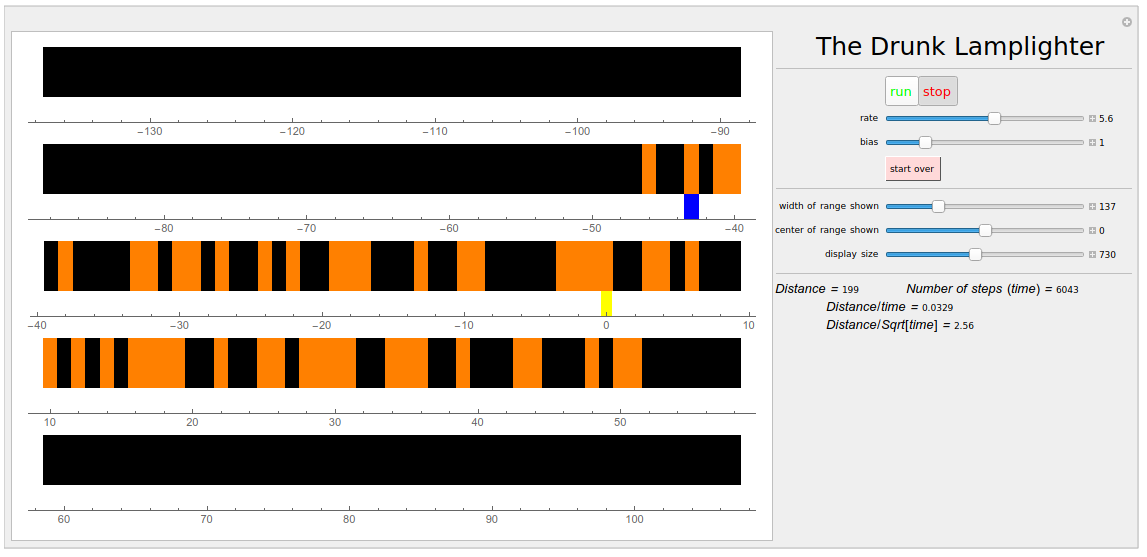Back to top of page Privacy policyView My Stats